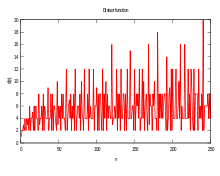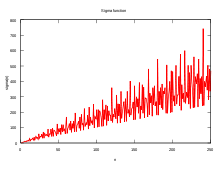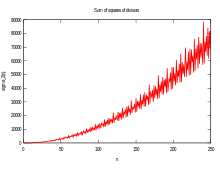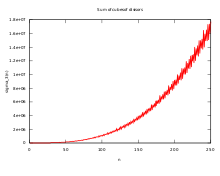# Divisor function

"Robin's theorem" redirects here. For Robbins' theorem in graph theory, see Robbins' theorem.Divisor function σ0(n) up to n = 250Sigma function σ1(n) up to n = 250Sum of the squares of divisors, σ2(n), up to n = 250Sum of cubes of divisors, σ3(n) up to n = 250

In mathematics, and specifically in number theory, a divisor function is an arithmetic function related to the divisors of an integer. When referred to as the divisor function, it counts the number of divisors of an integer. It appears in a number of remarkable identities, including relationships on the Riemann zeta function and the Eisenstein series of modular forms. Divisor functions were studied by Ramanujan, who gave a number of important congruences and identities; these are treated separately in the article Ramanujan's sum.

A related function is the divisor summatory function, which, as the name implies, is a sum over the divisor function.

## Definition

The sum of positive divisors function σx(n), for a real or complex number x, is defined as the sum of the xth powers of the positive divisors of n. It can be expressed in sigma notation aswhereis shorthand for "d divides n". The notations d(n), ν(n) and τ(n) (for the German Teiler = divisors) are also used to denote σ0(n), or the number-of-divisors function (). When x is 1, the function is called the sigma function or sum-of-divisors function, and the subscript is often omitted, so σ(n) is the same as σ1(n) ().

The aliquot sum s(n) of n is the sum of the proper divisors (that is, the divisors excluding n itself, ), and equals σ1(n)  n; the aliquot sequence of n is formed by repeatedly applying the aliquot sum function.

## Example

For example, σ0(12) is the number of the divisors of 12:while σ1(12) is the sum of all the divisors:and the aliquot sum s(12) of proper divisors is:## Table of values

n Divisors σ0(n) σ1(n) s(n) = σ1(n)  n Comment
1 1 1 1 0 square number: σ0(n) is odd; power of 2: s(n) = n  1 (almost-perfect)
2 1, 2 2 3 1 Prime: σ1(n) = 1 + n so s(n) = 1; power of 2: s(n) = n  1 (almost-perfect)
3 1, 3 2 4 1 Prime: σ1(n) = 1 + n so s(n) = 1
4 1, 2, 4 3 7 3 square number: σ0(n) is odd; power of 2: s(n) = n  1 (almost-perfect)
5 1, 5 2 6 1 Prime: σ1(n) = 1 + n so s(n) = 1
6 1, 2, 3, 6 4 12 6 first perfect number: s(n) = n
7 1, 7 2 8 1 Prime: σ1(n) = 1 + n so s(n) = 1
8 1, 2, 4, 8 4 15 7 power of 2: s(n) = n 1 (almost-perfect)
9 1, 3, 9 3 13 4 square number: σ0(n) is odd
10 1, 2, 5, 10 4 18 8
11 1, 11 2 12 1 Prime: σ1(n) = 1 + n so s(n) = 1
12 1, 2, 3, 4, 6, 12 6 28 16 first abundant number: s(n) > n
13 1, 13 2 14 1 Prime: σ1(n) = 1 + n so s(n) = 1
14 1, 2, 7, 14 4 24 10
15 1, 3, 5, 15 4 24 9
16 1, 2, 4, 8, 16 5 31 15 square number: σ0(n) is odd; power of 2: s(n) = n  1 (almost-perfect)
σ0(n) +0 +1 +2 +3 +4 +5 +6 +7 +8 +9 +10 +11
0+ 1 2 2 3 2 4 2 4 3 4 2
12+ 6 2 4 4 5 2 6 2 6 4 4 2
24+ 8 3 4 4 6 2 8 2 6 4 4 4
36+ 9 2 4 4 8 2 8 2 6 6 4 2
48+ 10 3 6 4 6 2 8 4 8 4 4 2
60+ 12 2 4 6 7 4 8 2 6 4 8 2
72+ 12 2 4 6 6 4 8 2 10 5 4 2
84+ 12 4 4 4 8 2 12 4 6 4 4 4
96+ 12 2 6 6 9 2 8 2 8 8 4 2
108+ 12 2 8 4 10 2 8 4 6 6 4 4
120+ 16 3 4 4 6 4 12 2 8 4 8 2
132+ 12 4 4 8 8 2 8 2 12 4 4 4
σ1(n) +0 +1 +2 +3 +4 +5 +6 +7 +8 +9 +10 +11
0+ 1 3 4 7 6 12 8 15 13 18 12
12+ 28 14 24 24 31 18 39 20 42 32 36 24
24+ 60 31 42 40 56 30 72 32 63 48 54 48
36+ 91 38 60 56 90 42 96 44 84 78 72 48
48+ 124 57 93 72 98 54 120 72 120 80 90 60
60+ 168 62 96 104 127 84 144 68 126 96 144 72
72+ 195 74 114 124 140 96 168 80 186 121 126 84
84+ 224 108 132 120 180 90 234 112 168 128 144 120
96+ 252 98 171 156 217 102 216 104 210 192 162 108
108+ 280 110 216 152 248 114 240 144 210 182 180 144
120+ 360 133 186 168 224 156 312 128 255 176 252 132
132+ 336 160 204 240 270 138 288 140 336 192 216 168
σ2(n) +0 +1 +2 +3 +4 +5 +6 +7 +8 +9 +10 +11
0+ 1 5 10 21 26 50 50 85 91 130 122
12+ 210 170 250 260 341 290 455 362 546 500 610 530
24+ 850 651 850 820 1050 842 1300 962 1365 1220 1450 1300
36+ 1911 1370 1810 1700 2210 1682 2500 1850 2562 2366 2650 2210
48+ 3410 2451 3255 2900 3570 2810 4100 3172 4250 3620 4210 3482
60+ 5460 3722 4810 4550 5461 4420 6100 4490 6090 5300 6500 5042
72+ 7735 5330 6850 6510 7602 6100 8500 6242 8866 7381 8410 6890
84+ 10500 7540 9250 8420 10370 7922 11830 8500 11130 9620 11050 9412
96+ 13650 9410 12255 11102 13671 10202 14500 10610 14450 13000 14050 11450
108+ 17220 11882 15860 13700 17050 12770 18100 13780 17682 15470 17410 14500
120+ 22100 14763 18610 16820 20202 16276 22750 16130 21845 18500 22100 17162
132+ 25620 18100 22450 21320 24650 18770 26500 19322 27300 22100 25210 20740

## Properties

For a non-square integer, n, every divisor, d, of n is paired with divisor n/d of n andis even; for a square integer, one divisor (namely) is not paired with a distinct divisor andis odd. Similarly, the numberis odd if and only if n is a square or twice a square.

For a prime number p,because by definition, the factors of a prime number are 1 and itself. Also, where pn# denotes the primorial,since n prime factors allow a sequence of binary selection (or 1) from n terms for each proper divisor formed.

Clearly,and σ(n) > n for all n > 2.

The divisor function is multiplicative, but not completely multiplicative. The consequence of this is that, if we writewhere r = ω(n) is the number of distinct prime factors of n, pi is the ith prime factor, and ai is the maximum power of pi by which n is divisible, then we havewhich is equivalent to the useful formula:It follows (by setting x = 0) that d(n) is:For example, if n is 24, there are two prime factors (p1 is 2; p2 is 3); noting that 24 is the product of 23×31, a1 is 3 and a2 is 1. Thus we can calculateas so:The eight divisors counted by this formula are 1, 2, 4, 8, 3, 6, 12, and 24.

We also note s(n) = σ(n)  n. Here s(n) denotes the sum of the proper divisors of n, that is, the divisors of n excluding n itself. This function is the one used to recognize perfect numbers which are the n for which s(n) = n. If s(n) > n then n is an abundant number and if s(n) < n then n is a deficient number.

If n is a power of 2, for example,, thenand s(n) = n - 1, which makes n almost-perfect.

As an example, for two distinct primes p and q with p < q, letThenandwhere φ(n) is Euler's totient function.

Then, the roots of:allow us to express p and q in terms of σ(n) and φ(n) only, without even knowing n or p+q, as:Also, knowing n and either σ(n) or φ(n) (or knowing p+q and either σ(n) or φ(n)) allows us to easily find p and q.

In 1984, Roger Heath-Brown proved that the equalityis true for an infinity of values of n.

## Series relations

Two Dirichlet series involving the divisor function are:which for d(n) = σ0(n) givesandA Lambert series involving the divisor function is:for arbitrary complex |q|  1 and a. This summation also appears as the Fourier series of the Eisenstein series and the invariants of the Weierstrass elliptic functions.

## Growth rate

In little-o notation, the divisor function satisfies the inequality (see page 296 of Apostol’s book)More precisely, Severin Wigert showed thatOn the other hand, since there are infinitely many prime numbers,In Big-O notation, Peter Gustav Lejeune Dirichlet showed that the average order of the divisor function satisfies the following inequality (see Theorem 3.3 of Apostol’s book)whereis Euler's gamma constant. Improving the boundin this formula is known as Dirichlet's divisor problem

The behaviour of the sigma function is irregular. The asymptotic growth rate of the sigma function can be expressed by:where lim sup is the limit superior. This result is Grönwall's theorem, published in 1913 (Grönwall 1913). His proof uses Mertens' 3rd theorem, which says thatwhere p denotes a prime.

In 1915, Ramanujan proved that under the assumption of the Riemann hypothesis, the inequality:(Robin's inequality)

holds for all sufficiently large n (Ramanujan 1997). The largest known value that violates the inequality is n=5040. In 1984 Guy Robin proved that the inequality is true for all n > 5040 if and only if the Riemann hypothesis is true (Robin 1984). This is Robin's theorem and the inequality became known after him. Robin furthermore showed that if the Riemann hypothesis is false then there are an infinite number of values of n that violate the inequality, and it is known that the smallest such n > 5040 must be superabundant (Akbary & Friggstad 2009). It has been shown that the inequality holds for large odd and square-free integers, and that the Riemann hypothesis is equivalent to the inequality just for n divisible by the fifth power of a prime (Choie et al. 2007).

Robin also proved, unconditionally, that the inequalityholds for all n ≥ 3.

A related bound was given by Jeffrey Lagarias in 2002, who proved that the Riemann hypothesis is equivalent to the statement thatfor every natural number n > 1, whereis the nth harmonic number, (Lagarias 2002).